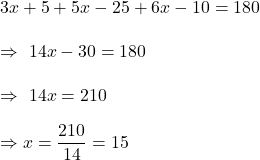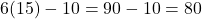## The measures of the base angles of an isosceles triangle are 3x 5 and 5x – 25. The measure of the vertex angle is 6x – 10. Find the measur

Question

The measures of the base angles of an isosceles triangle are 3x 5 and 5x – 25. The measure of the vertex angle is 6x – 10. Find the measure of the vertex angle.

in progress 0
5 months 2021-08-09T14:16:17+00:00 1 Answers 5 views 0

1. Answer: The measure of the vertex angle = 80°

Step-by-step explanation:

Angle sum property of triangle : The sum of the measures of the angles for any triangle is 180 degrees.

So,Then, vertex angle =Hence, The measure of the vertex angle = 80°